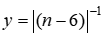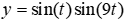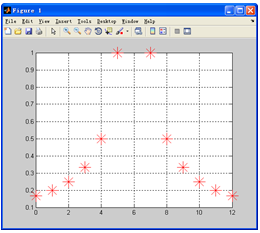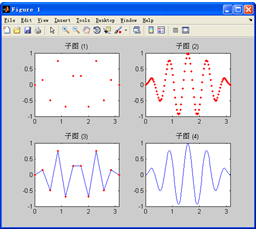# MATLAB数据可视化

### 数据可视化

（1）交互性：用户可以方便地以交互的方式管理和开发数据。

（2）多维性：可以看到表示对象或事件的数据的多个属性或变量，而数据可以按其每一维的值，将其分类、排序、组合和显示。

（3）可视化：数据可以用图像、曲线、二维图形、三维图形和动画等来显示，并可对其模式和相互关系进行可视化分析。

7.1  绘图的基本知识

7.1.1  离散数据和离散函数的可视化

【例7-1】  用图形表示离散函数。Ex_7_1.m

n=0:12;

y=1./abs(n-6);                              %  准备离散点数据

plot(n,y,’r*’,’MarkerSize’,20)           %  绘图

grid on

7.1.2  连续函数的可视化

【例7-2】  用图形表示连续调制波形。Ex_7_2.m

t1=(0:11)/11*pi;              %  自变量

y1=sin(t1).*sin(9*t1);        %  对应的函数值

t2=(0:100)/100*pi;

y2=sin(t2).*sin(9*t2);

%  以下为绘图，将几个图作为子图放在一起便于比较

subplot(2,2,1),plot(t1,y1,’r.’),axis([0,pi,-1,1]),title(‘子图 (1)’)

subplot(2,2,2),plot(t2,y2,’r.’),axis([0,pi,-1,1]),title(‘子图 (2)’)

subplot(2,2,3),plot(t1,y1,t1,y1,’r.’)

axis([0,pi,-1,1]),title(‘子图 (3)’)

subplot(2,2,4),plot(t2,y2)

axis([0,pi,-1,1]),title(‘子图 (4)’)7.1.3  可视化的一般步骤

 步    骤 典型命令 1．数据准备 选定所要绘图的范围 产生自变量采样向量 计算相应的函数值向量 t=pi*(0:100)/100 y=sin(t).*sin(9*t); 2．选定图形窗口及子图位置 默认打开Figure. 1，或当前窗口，或当前子图。可以用命令指定图形窗口和子图位置 figure(1) subplot(2,2,3) 3．调用绘图命令（可以包括线型、色彩、数据点型） plot(t,y,‘b-’)      %   用蓝色实线绘图 4．设置轴的范围与刻度、坐标网格 axis([0,pi,-1,1])    %  设置轴的范围 grid on           %  画坐标网格 5．图形注释： 图名、坐标名、图例、文字说明等 title(‘figure’)        %   图名 xlabel(‘t’); ylabel(‘y’)  %  轴名 legend(‘sin(t)’, ‘sin(t)。*sin(9*t)’)  %  图例 text(2,0.5,‘y=sin(t).*sin(9*t)’)% 文字说明 6．图形的精细修饰 利用对象属性值设置，利用图形窗口工具栏设置 set(h,‘MarkerSize’,10) %  设置数据点大小 7．图形的导出与打印 %  采用图形窗口菜单操作